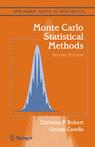## Typo in Example 5.18Edward Kao is engaged in a detailed parallel reading of Monte Carlo Statistical Methods and of Introducing Monte Carlo Methods with R. He has pointed out several typos in Example 5.18 of Monte Carlo Statistical Methods which studies a missing data phone plan model and its EM resolution. First, the customers in area i should be double-indexed, i.e.$Z_{ij}\sim\mathcal{M}(1,(p_1,\ldots,p_5))$

which implies in turn that$T_i=\sum_{j=1}^{n_j}Z_{ij}$.

Then the summary T should be defined as$\mathbf{T}=(T_1,T_2,\ldots,T_n)$

and$W_5$ as$W_5=\sum_{i=m+1}^nT_{i5},$

given that the first m customers have the fifth plan missing.

This site uses Akismet to reduce spam. Learn how your comment data is processed.